### Home > CALC > Chapter 1 > Lesson 1.4.4 > Problem1-181

1-181.
1. The graph below represents velocity of an object as a function of time. Trace it on your paper. Homework Help ✎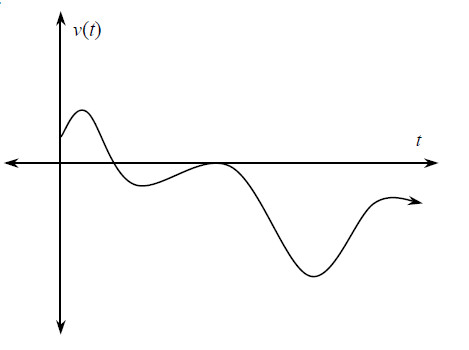1. Put a star * at the point where velocity is the greatest.

2. With another color, sketch a graph of the speed on the same set of axes.

3. Indicate with a double star ** the position where speed is the greatest.

4. Explain why the greatest velocity and the greatest speed do not occur at the same position.

5. Sketch a new graph where speed and velocity have the same maximum value.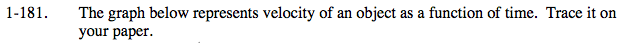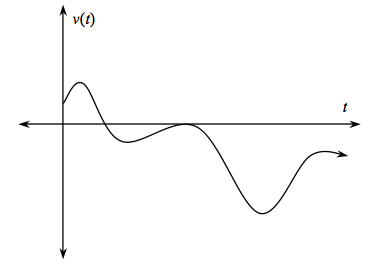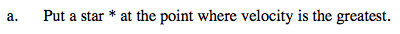What is the maximum point on the graph?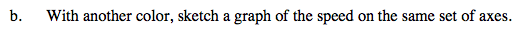Speed is the absolute value of velocity. Flip all the negative y-values to their corresponding positive positions.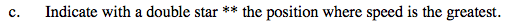What is the maximum point on the speed graph? Notice that this is NOT the same as the maximum point on the velocity graph.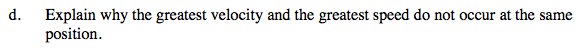The maximum is the greatest positive value. How does changing the graph to an absolute value graph change the possible maximum?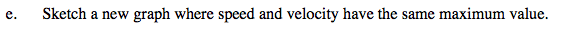A correct velocity graph will have a maximum above the x-axis and a minimum below the x-axis, but the maximum point is further from the x-axis than the minimum point. In other words, |maximum| > |minimum|.